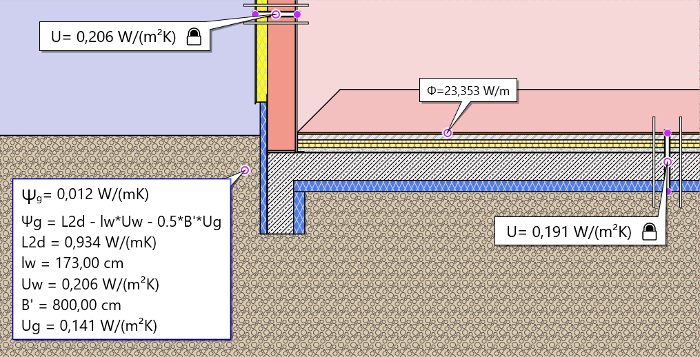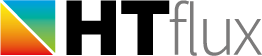# Ψg-calculation with slab on or near the grade level according to ISO 10211 and ISO 13370

This method is used for slabs that are located on or near the surface level of the ground. Generally this method should be used when the energy loss of the detail is described by using a single U-value for the wall and the Ug value for the floor. With this version HTflux will do the calculation of Ug according to the method described in ISO 13370. This value should match with the one you are using for your building energy performance. If for some reason you want to preset a given Ug value, instead you can use the manual input method.
The Ψg value calculated with this method will compensate for the wall’s contact to the ground, the actual slab edge heat flux and the heat flux through the floor slab itself.

The Ψg value will be calculated by measuring the heat-flux of the model and subtracting the heat-fluxes described by U-values afterwards: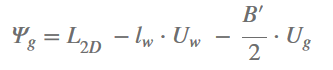The calculation is therefore very similar to other Ψ value calculations. The important difference is that you will have to use the Ug value for the floor slab heat flux and not the U-value of the floor slab itself. This adapted value is based on the U-value of the slab, but also contains the thermal resistance of the ground itself. Based on the slab dimension and U value of the floor an effective thickness for the ground will be calculated. Since the Ug value of is also considering the insulating effect of the ground below, it will always be lower than the U value of the floor slab itself. (more information on this can be found in the  standard ISO 13370.)
Since the Ψg calculation will also consider the difference between the actual floor slab heat flux and the heat flux as described by Ug, it is important to perform the simulation using the correct width of the model (B’/2).  It will only be valid for this width. However for larger floor slabs the ISO standard defines a limit: if you perform the calculation for a model with a characteristic floor slab dimension of 8m (=4m model width), the resulting Ψg can be used for any slabs larger than this also.

## Detailed work-flow

### 1) calculate the characteristic floor slab dimension.

see characteristic floor slab dimension for reference.

### 2) draw the model of the slab edge

Draw a model of your slab edge width that equals B’/2. Make sure to use the appropriate dimensional reference.
If B’ exceeds 8m you should always use a width of 4m for the model. To the top side you should draw at least 1m of the wall measured from the top of the floor or ground-level (whatever is higher) to comply with the rules in ISO 10211. You might have additional elements in your wall in this area, in this case you should draw up to 1m of the homogeneous wall above this region.

Add a block of soil with a width and height of 2,5 x B’ to your model. If this value exceeds 20m use 20m instead.
Use the correct material for the soil block. The standard ISO 13370 recommends to use

• clay or silt
• sand or gravel
• solid rock

depending on the actual soil material. Note: To easily find these materials in the HTflux database you can either select “soil” in the category filter or type in “13370” in the text search.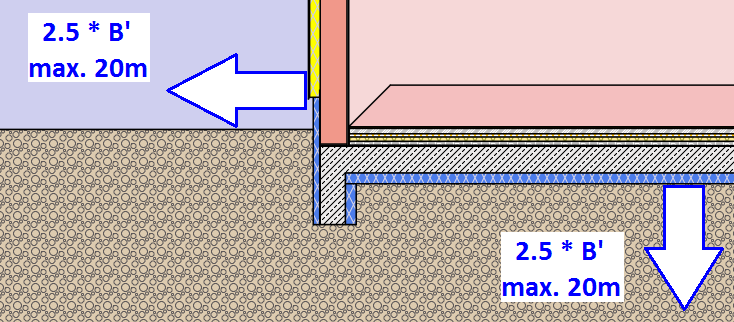### 4) select correct boundary conditions

use a boundary condition with R = 0,04 m²K/W for the external climate, R=0,17 m²K/W for the internal floor and R=0,13 m²K/W for the internal wall surface.
(see boundary conditions and surface resistances for reference). You can do the calculation with any internal/external temperature combination, as you will always get the same results.

### 5) add a heat-flux measurement tool

Add a heat-flux measurement tool and configure it to measure the total heat-flux from the internal surfaces. (see heat flux tool)

### 6) add U value tools

Apply a U-value tool for the wall and one for the floor. To be able to use the U-value tool for the floor, the soil material has to be replaced by a boundary condition temporarily. Therefore use or create a boundary condition for the soil, with a weighted temperature type (e.g. use 0,50 or 0,80) and make sure that the heat transfer resistance is set to 0,00.

### 7) run the simulation with the soil boundary condition

if you have replaced the soil material with the soil boundary conditions, now both U-tools should display correct U-values.
Double-click on the U-value tags and configure correctly: make sure to select the 1d-U value and use the lock symbol on the top right side to freeze the tool. That way you will be able to use the U-values calculated later on.

### 8) re-run simulation with soil material

Replace the boundary condition of the soil block with the soil material (see step 3). Re-run the simulation. Make sure to use the highest possible resolution for the simulation. If you are limited to 8GB RAM you should use 10mm (or less), if you have 16GB RAM you should use 5mm (or less) for this step. This will ensure the best quality for the calculation results.

### 9) add and configure a Ψg calculation tool

Use the Ψg-button to add a new calculation, pick the option “ISO 10211/12270, slab on grade” for this calculation.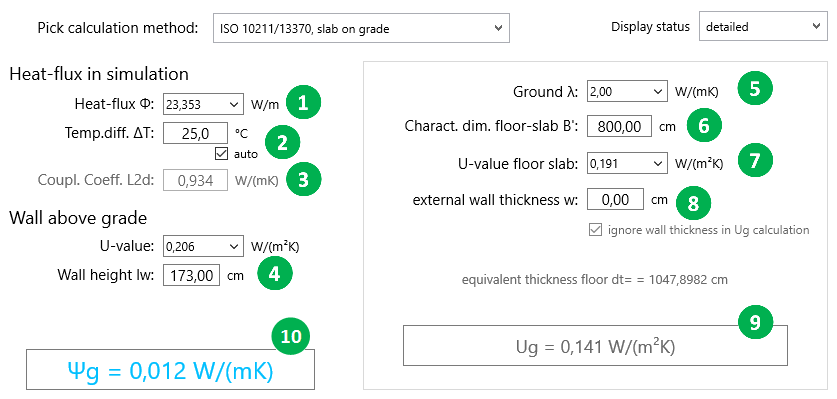1. Pick the heat-flux measurement tool for the internal heat-flux here (use the drop-down list if you have applied more tools).
2. Here you can enter the temperature difference relevant for the heat-flux. Usually this will be the internal/external temperature difference. In this case you should leave the “auto” option turn on and you will not have to input a value here.
3. The calculated L2D value is displayed here. (heat-flux divided by temperature difference)
4. Use the drop-down list to select the U-Value of the wall. (see step 6)
Make sure you enter the correct length for the wall here. Measure this length from the top of your simulation region down to your bottom reference level (see dimensional reference)
5. Enter the conductivity of the soil material used here. This is necessary for the correct Ug calculation.
6. Enter the characteristic dimension of the floor slab B’. This value should correspond with the double width of your model.
7. Use the drop down-list to select the U-Value that corresponds with your floor slab. (see step 6)
8. Here you can type in the thickness of an external wall. The value will affect the calculation. However most set this thickness to “0”, as it assumes an external wall beside the floor slab. Anyway you should choose the method inline with your building performance calculation.
9. The resulting Ug value is displayed here. (above this field you will find the equivalent thickness of the floor used in the calculation, for more information please refer to ISO 13370).
10. If all necessary inputs are provided the calculation result for the Ψg value will be displayed here.

The calculation is now ready. If you close the dialog, the results along with the forumula and calculation parameters used will be displayed in the according measurement tag. This will make the calculation transparent and traceable. You can arrange the results and use the report tools to create a report of your calculation.